Search by Topic

Filter by: Content type:
Age range:
Challenge level:

There are 11 results

Broad Topics > Coordinates and Coordinate Geometry > Cartesian equations of lines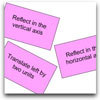Surprising Transformations

Age 14 to 16 Challenge Level:

I took the graph y=4x+7 and performed four transformations. Can you find the order in which I could have carried out the transformations?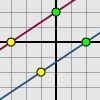Translating Lines

Age 11 to 14 Challenge Level:

Investigate what happens to the equation of different lines when you translate them. Try to predict what will happen. Explain your findings.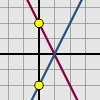Reflecting Lines

Age 11 to 14 Challenge Level:

Investigate what happens to the equations of different lines when you reflect them in one of the axes. Try to predict what will happen. Explain your findings.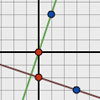Perpendicular Lines

Age 14 to 16 Challenge Level:

Position the lines so that they are perpendicular to each other. What can you say about the equations of perpendicular lines?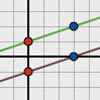Parallel Lines

Age 11 to 14 Challenge Level:

How does the position of the line affect the equation of the line? What can you say about the equations of parallel lines?Enclosing Squares

Age 11 to 14 Challenge Level:

Can you find sets of sloping lines that enclose a square?Kite in a Square

Age 14 to 16 Challenge Level:

Can you make sense of the three methods to work out the area of the kite in the square?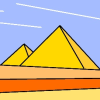Painting Between the Lines

Age 11 to 16 Challenge Level:

In abstract and computer generated art, a real object can be represented by a simplified set of lines. Can you create a picture using mathematical instructions?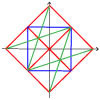Coordinate Designs

Age 11 to 14 Challenge Level:Close to Triangular

Age 14 to 16 Challenge Level:

Drawing a triangle is not always as easy as you might think!Reflect Again

Age 16 to 18 Challenge Level:

Follow hints to investigate the matrix which gives a reflection of the plane in the line y=tanx. Show that the combination of two reflections in intersecting lines is a rotation.